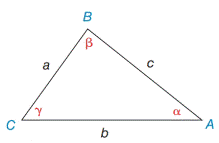Chapter 11.4, Problem 1EElementary Geometry For College St...

7th Edition
Alexander + 2 others
ISBN: 9781337614085

Solutions

Chapter
SectionElementary Geometry For College St...

7th Edition
Alexander + 2 others
ISBN: 9781337614085
Textbook Problem

In Exercises 1 and 2, use the given information to find an expression for the area of Δ A B C . Give the answer in a form such as A = 1 2 ( 3 ) ( 4 )  sin 32 ∘ . See the figure for Exercises 1 to 8.a) a = 5 ,   b = 6 ,  and  γ = 78 ∘ b) a = 5 ,   b = 7 ,   α = 36 ∘  and  β = 88 ∘Exercises 1-8

To determine

a)

To find:

The area of the ABC in the form of A=12(a)(b) sin γ

If a=5, b=6, and γ=78

Explanation

1) Formula:

The area of an acute triangle equals one-half the product of the lengths of two sides and the sine of the included angle.

The area of the triangle ABC is given by,

A=12absinγ

2) Calculation:

Given,

In triangle ABC,

a=5b=6 andγ=78

The area of the triangle ABC A=12absinγ

To determine

b)

To find:

The area of the ABC in the form of A=12(a)(b) sin β

If a=5, b=7, α=36 and β=88

Still sussing out bartleby?

Check out a sample textbook solution.

See a sample solution

The Solution to Your Study Problems

Bartleby provides explanations to thousands of textbook problems written by our experts, many with advanced degrees!

Get Started

Explain the difference between a matched-subjects design and a repeated-measures design.

Essentials of Statistics for The Behavioral Sciences (MindTap Course List)

Evaluate the integral. 16. 02/2x21x2dx

Single Variable Calculus: Early Transcendentals

The fourth term of {an} defined by a1 = 3, and for n = 1, 2, 3, … is:

Study Guide for Stewart's Single Variable Calculus: Early Transcendentals, 8th

, where E is the wedge-shaped solid shown at the right, equals:

Study Guide for Stewart's Multivariable Calculus, 8th